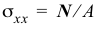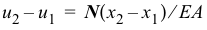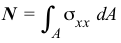# 3.1 – THEORY OF AXIAL MEMBERS

 div.mlw_qmn_quiz input[type=radio], div.mlw_qmn_quiz input[type=submit], div.mlw_qmn_quiz label { cursor: pointer; } div.mlw_qmn_quiz input:not([type=submit]):focus, div.mlw_qmn_quiz textarea:focus { background: #eaeaea; } div.mlw_qmn_quiz { text-align: left; } div.quiz_section { } div.mlw_qmn_timer { position:fixed; top:200px; right:0px; width:130px; color:#00CCFF; border-radius: 15px; background:#000000; text-align: center; padding: 15px 15px 15px 15px } div.mlw_qmn_quiz input[type=submit], a.mlw_qmn_quiz_link { border-radius: 4px; position: relative; background-image: linear-gradient(#fff,#dedede); background-color: #eee; border: #ccc solid 1px; color: #333; text-shadow: 0 1px 0 rgba(255,255,255,.5); box-sizing: border-box; display: inline-block; padding: 5px 5px 5px 5px; margin: auto; } In the questions below, select the correct answer. Name Email 1.  Axial strain is uniform across a nonhomogeneous cross section. True False 2.  Axial stress is uniform across a nonhomogeneous cross section. True False 3.  The formulacan be used for finding the stress on a cross section of a tapered axial member. True False 4.  The formulacan be used for finding the deformation of a segment of a tapered axial member. True False 5.  The formulacan be used for finding the stress on a cross section of an axial member subjected to distributed forces. True False 6.  The formulacan be used for finding the deformation of a segment of an axial member subjected to distributed forces. True False 7.  The equationcannot be used for nonlinear materials. True False 8.  The equationcan be used for a nonhomogeneous cross section. True False 9.  External axial forces must be colinear and pass through the centroid of a homogeneous cross section for no bending to occur. True False 10.  Internal axial forces jump by the value of the concentrated external axial force at a section. True False Do you have any comments or suggestions? Please send them to author@madhuvable.orgTime's up